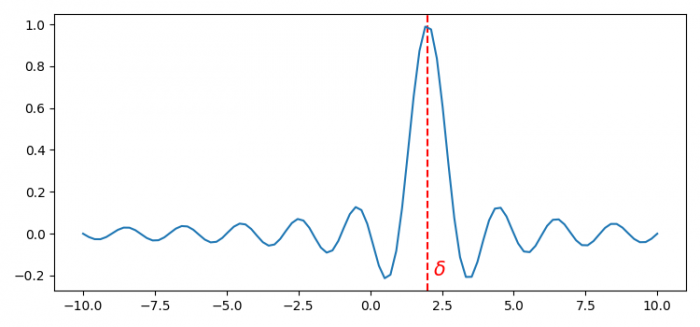# How can I move a tick label without moving corresponding tick in Matplotlib?

To move a tick label without moving corresponding tick in Matplotlib, we can use axvline() method and can annotate it accordingly.

## Steps

• Set the figure size and adjust the padding between and around the subplots.
• Initialize a variable, delta.
• Create x and y data points using numpy.
• Plot delta using axvline() method
• Annotate that line using annotate() method.
• Plot x and y data points using plot() method.
• To display the figure, use show() method.

## Example

from matplotlib import pyplot as plt
import numpy as np

plt.rcParams["figure.figsize"] = [7.50, 3.50]
plt.rcParams["figure.autolayout"] = True

delta = 2.0
x = np.linspace(-10, 10, 100)
y = np.sinc(x - delta)

plt.axvline(delta, ls="--", color="r")
plt.annotate(r"$\delta$", xy=(delta + 0.2, -0.2), color="r", size=15)
plt.plot(x, y)

plt.show()

## Output